# SQL – Postgresql Indexing

Indexing is a technique upon the designation of databases. It is intended to, hopefully, make queries faster. While technical details of Index might be different for different types of databases, this post attempts to give a comprehensive view of this component for Postgresql specifically.

#### Table of content

Intro to Index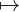What is Indexing?Goods and BadsGuidelinesIndex Types
Indexing commandsSimple IndexMulti-column IndexPartial IndexIndex-only ScansUnique-Index

## Intro to Index

### What is Indexing?

Databases with and without indexes can be thought of as sorted and unsorted arrays, respectively.

Imagine we make a query:

SELECT x FROM tb WHERE y > 3;

Without prior-indexing, the system will have to scan the whole table tb, check each record to see if its y-column is larger than 3, if it is, fetches its x-column’s value. This requires a whole-table search.

However, if prior to the query, we created an index on the y column, by running a command such as:

CREATE INDEX table_tb_index_y ON tb (y);

The system would have created a sorted index of table tb based on column y and saved this index in a separate place, so that we have the index to utilize when necessary. Look at the below figure for an intuitive illustration.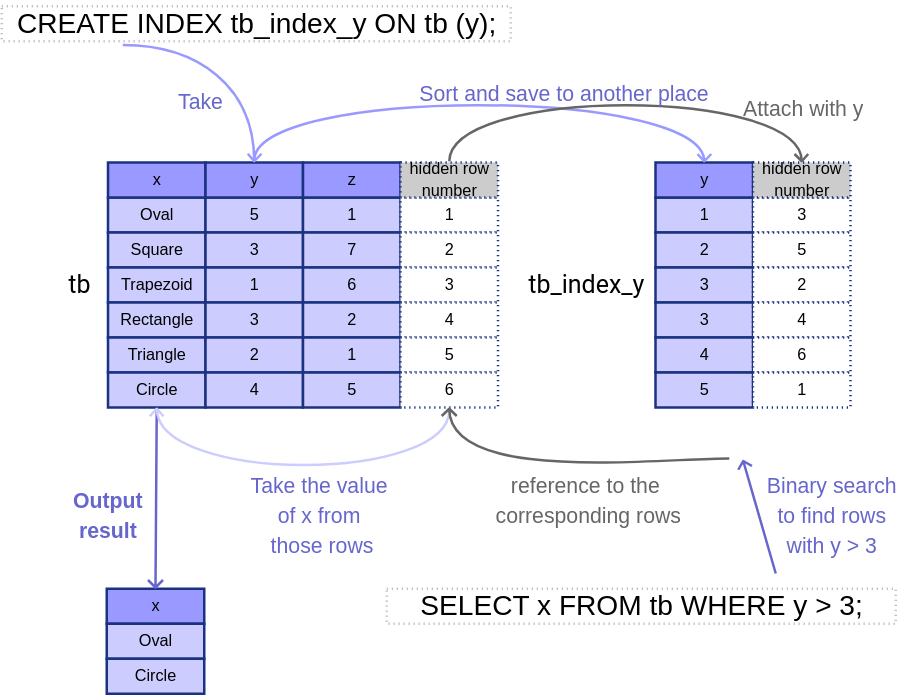Illustration of Indexing. In this example, for simplification, an Index is represented by a sorted array. In practice, the most common Index structure is the B-tree, a generalization of the Binary-Search Tree.

The simplest command to create index follows the format:

CREATE INDEX index_name ON table (column);

while dropping an index is as straightforward as:

DROP INDEX index_name;

Note that the application of indexes on clients’ commands is automatic, i.e. we are the ones who create the indexes, after that, the system will choose to use which index (or no index) to utilize for future commands on its own. The only exception for this rule is: the system itself automatically creates an index for every primary key and uniquely-defined column.

The index has the potential to speed up queries as shown in the above example when an O(n) scan can be replaced by an O(log n) binary search. Note that we always need an additional O(result-size) complexity for fetching the results.

The commands that might benefit from indexes are: SELECT, UPDATE, JOIN, and DELETE.

Nevertheless, it comes with a cost of pre-computation: the system must have scanned, sorted the indexes at the beginning, and a memory overhead: we need space to save the indexes. Furthermore, every time there is a change in the table (e.g. UPDATE, INSERT), the corresponding indexes must also be processed.

It is also worth it to mention that while indexes might reduce the complexity from O(n) to O(log n), they also increase the constant factor k due to their handling of metadata and references. Thus, when n is small, using an index may even increase the processing time.

### Guidelines

While creating indexes is a trade-off, here are some guidelines on when we should take advantage of this feature:

• Only create indexes for big tables. For small tables, the increase in constant factor might make queries-with-indexes slower than query-without-indexes.
• When the number of retrieval commands is much higher than the number of manipulations. Because we benefit from retrieving with indexes and we have to pay the cost when manipulating rows. Of course, this is only applied for the commands and indexes that are in relation to each other, a command that only involves the column u has almost nothing to do with an index on the column v.
• The outputs for the retrieval commands should be small. When the output contains a large number of rows, the system has to travel a lot from the index to the original table to collect results, which makes it ineffective. In fact, it is stated that when a query searches for a common value (one that accounts for more than a few percentages of the table), indexes will not be used.
• The columns that are indexes should have a small number of Nulls. By default, the system does not index Null values. Hence, when a column is dominated by Nulls, indexing it has little effect on the overall process.
• Consider adding indexes for foreign keys if needed, this may optimize the performance related to referential linking.
• The more the column’s values are unique, the more the index’s strength. For example, if the column is binary with approximately 50% of 0 and 50% of 1, an index on this column can at most filter out only a half of the rows.

### Index Types

Postgresql supports 6 types of index structure.

• The B-tree index is the default, which can handle equality and range queries. Some operators that can invoke the use of the B-tree index are: =, >, <.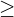,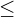, BETWEEN, IN, LIKE ‘bar%’ (i.e. regular expression of strings starting with ‘bar’). B-tree is also the only index type that has innate sorting of results.
• Hash index only supports equality.
• The GIST index is not a single kind of index, but rather a collection of many indexing strategies. GIST is often used for 2-D geometric data types.
• The SP-GIST index is, similar to GIST, an infrastructure of many indexing strategies. The standard distribution of Postgresql includes SP-GIST operator classes for 2-d points.
• The GIN index also supports many different user-defined search schemes. However, GIN is mostly used for indexing columns of array-type.
• The BRIN index saves summaries of the values in consecutive physical block ranges of a table.

To explicitly specify the indexing method for an index, we add the term USING method:

CREATE INDEX index_name ON table USING method (col);

## Indexing commands

### Simple index

A normal indexing command takes this form:

CREATE INDEX index_name ON table (column/expression);

While creating an index on a column is trivial and has been mentioned above, making one with an expression is also supported.

Example 1: Create an index with the lower case of a column containing strings.

CREATE INDEX tb_lower_job_idx ON tb (lower(job));

Example 2: If clients often query information by full names of people:

CREATE INDEX people_fullname_idx ON people ((first_name || '  ' || last_name));

### Multi-column index

Basically, a multi-column index takes this form:

CREATE INDEX index_name ON table (col1, col2, col3);

Property 1:

For this multi-column indexing, a comparison between 2 rows first compares their values on col1, if those are equal, compare their values on col2, if they are still equal, values on col3 are compared. Hence, the order col1, col2, col3 is very important, which is completely different from col3, col1, col2 and even col1, col3, col2.

When querying on these 3 indexed columns, we should also keep their order to be col1, col2, col3. If, for example, we only want to query on col1 and col3, stating the order as col1, col3 will trigger the indexing while col3, col1 will not.

Suppose we declare an index rule as below:

CREATE INDEX t_abc_idx ON t (a, b, c);

Queries on a, (a, b), (a, b, c) will take full use of the index (attention to the WHERE clause):

 SELECT * FROM t WHERE a = 3;
SELECT * FROM t WHERE a = 3 AND b = 'x';
SELECT * FROM t WHERE a = 3 AND b = 'x' AND c > 20;

The below query can also make use of this index:

 SELECT * FROM t WHERE a = 3 AND c < 10;

For this query, the system will use the index to locate all positions that have value a = 3, then it scans over all these rows to test for the condition c < 10.

For the below queries, this index is not applicable.

SELECT * FROM t WHERE b = 'z';
SELECT * FROM t WHERE b = 'u' AND c = 2 AND a = 100;
SELECT * FROM t WHERE c = 10 AND a = 10;

Property 2:

Instead of using a multi-column index, a multi-column conditioned query can also make use of several single indexes. For instance, suppose we declare the below 3 single indexes:

CREATE INDEX t_a_idx ON t (a);
CREATE INDEX t_b_idx ON t (b);
CREATE INDEX t_c_idx ON t (c);

And query:

SELECT * FROM t WHERE b = 'u' AND c = 2 AND a = 100;

The system can query 3 set: the first set of all rows that have b = ‘u’, this uses the second index; the second set of all rows that have c = 2, this uses the third index; and the third set of all rows that have a = 100, this uses the first index. Lastly, it applies the AND operator on these 3 sets to take only the intersection of them to return.

Property 3:

By default, when rows are sorted by an index, they are sorted by ascending order with Nulls being at the last positions. If we specify the order as descending, Nulls are space at the first positions by default. We also have the right to sort rows ascendingly with Nulls at first or descendingly with Nulls at last. Below illustrates the 4 possible setups.

CREATE INDEX t_a_idx ON t (a ASC NULLS FIRST);
CREATE INDEX t_a_idx ON t (a ASC NULLS LAST);
CREATE INDEX t_a_idx ON t (a DESC NULLS FIRST);
CREATE INDEX t_a_idx ON t (a DESC NULLS LAST);

This property does not greatly affect single indexing, however, for multi-column indexing with the ORDER BY clause, this might cause a problem. For example, an index with (a ASC, b ASC) would not be helpful with queries that need ODER BY (a ASC, b DESC) – these queries can only use indexes that are defined with (a ASC, b DESC) or (a DESC, b ASC).

### Partial Index

Partial Indexes are indexes that process on a subset of the table instead of on all rows. Use the WHERE clause to specify the conditions of rows to be indexed:

CREATE INDEX index_name ON table (column) WHERE condition;

Example: Suppose we have a table that contains both billed and unbilled orders, with the billed ones take the majority, however, the unbilled ones are of more interest and are more often queried. We may consider creating a partial index on unbilled orders such as:

CREATE order_unbilled_idx ON orders (order_id)
WHERE billed IS NOT TRUE;

The below queries may use this index:

SELECT * FROM orders WHERE billed IS NOT TRUE;
SELECT * FROM orders WHERE billed IS NOT TRUE AND amount > 1000;

### Index-only scans

Take a look at this example of a simple index from the beginning of the post.Illustration of Indexing. In this example, for simplification, an Index is represented by a sorted array. In practice, the most common Index structure is the B-tree, a generalization of the Binary-Search Tree.

As the query needs values on x-column, the system has to follow the references from the index to the original table to find and retrieve the results. However, if x-column is also attached to the index, the system will not have to travel back to the original table and that would save a lot of time. This is called an Index-only scan. To have the x-column on the index, we can either specify it in the create command (e.g. create an index on (y, x) instead of just on (x)) or just simply include x. Look at the figure below for the later solution.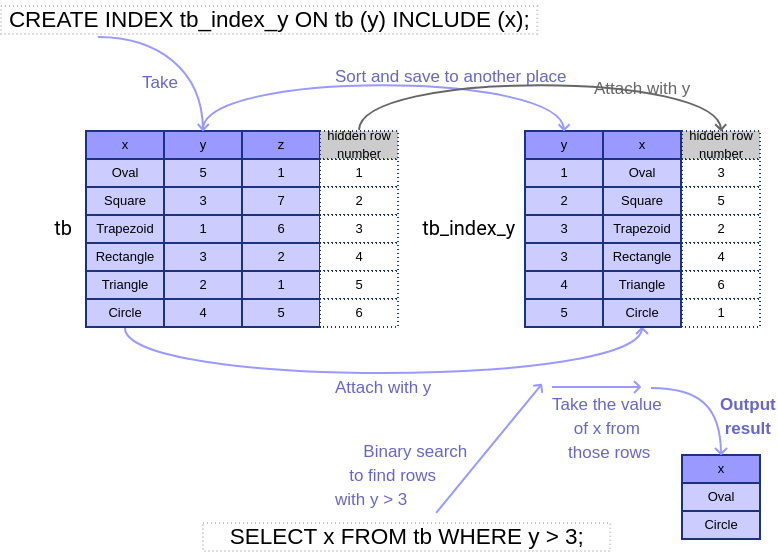Illustration of Index-only scanning. By including x on the index, the system needs not to travel back to the original data to retrieve the required results, which reduces processing time.

The clause to specify the attached column is INCLUDE:

CREATE INDEX index_name ON table (col1) INCLUDE (col2);

Property: An Index-only scan happens when the results to be queried exist in the index. However, in some more complex cases, there are some exceptions. For example, suppose we index an expression:

CREATE INDEX t_floor_a ON t (floor(a));

This following query, although seems to be feasible, actually requires the system to follow the references back to the table instead of doing an index-only scan:

SELECT floor(a) FROM t WHERE floor(a) < 10;

The reason is that the query requires outputs to be floor(a), which makes the system think that a need to be present in the index to be able to do an index-only scan. It doesn’t know that the presence of a is only needed to compute floor(a), which is already in the index.

For this example, adding INCLUDE x will make it work as expected.

### Unique-Index

An index can also be used to enforce the uniqueness of a column (or a combined set of columns).

CREATE UNIQUE INDEX index_name ON table (col1 [, col2, ...]);

References: